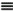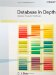# Some Equivalences

 I'll finish up this appendix with a few remarks on certain equivalences that might have already occurred to you. First, recall the IS_EMPTY operator, which I introduced in Chapter 5 and made much use of in Chapter 6. Well, if the system supports that operator, there's no logical need for it to support the quantifiers, thanks to the following equivalences: ` EXISTS x ( p)NOT ( IS_EMPTY ( p ) ) ` and: ` FORALL x ( p )IS_EMPTY ( p) ) ` In fact, the SQL support for quantifiers, such as it is, is based on exactly the foregoing equivalences. To be specific, SQL supports an EXISTS operator not really a quantifier as such, because it doesn't involve any bound variables that's defined as follows: the operator invocation EXISTS(tx), where tx is a table expression, returns TRUE if and only if the table t denoted by the expression tx is nonempty.[*] By contrast, SQL doesn't support a FORALL operator, because (a) we don't need both EXISTS and FORALL, and (b) more to the point, syntax of the form FORALL(tx) where tx is a table expression really makes no sense. For example, what could an expression like FORALL (SELECT * FROM S) possibly mean?[*] There's a certain irony here, though. As we saw in Chapter 3, because SQL supports nulls, it's based on what's called three-valued logic (instead of the conventional two-valued logic the relational model is based on). In three-valued logic, the existential quantifier can return three different results: TRUE, FALSE, and what's usually called UNKNOWN (as indeed it is, in SQL). But SQL's EXISTS operator always returns TRUE or FALSE, never UNKNOWN. As a consequence, (a) SQL's EXISTS is not a faithful implementation of the existential quantifier of three-valued logic, and (b) once again, SQL queries sometimes return the wrong answer. In a similar fashion, we don't absolutely need the quantifiers anyway if the system supports the aggregate operator COUNT. This is because of the following equivalences: ` EXISTS x ( p )COUNT ( p ) > 0 ` and: ` FORALL x ( p )COUNT ( p ) = COUNT ( x ) ` Now, I'm certainly not a fan of the idea of replacing quantified expressions by expressions involving COUNT invocations though sometimes we have to, if we're in a pure algebraic framework but it would be wrong of me not to mention the possibility.Database in Depth: Relational Theory for Practitioners
ISBN: 0596100124
EAN: 2147483647
Year: 2006
Pages: 127
Authors: C.J. Date

Similar book on Amazon